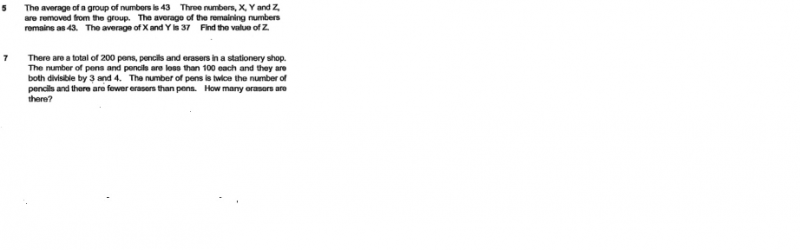# QuestionHi Kiasu Parents,

Thanks,

DNLNMUM

Source: 2018

If after the 3 numbers have been removed and the average of the remaining numbers remain at 43, this means the average of the 3 removed numbers is 43.

Total of X,Y and Z = 43 x 3 = 129

Total of X and Y = 37 x 2 = 74

Z = 129 – 74 = 55

Common multiples of 3 and 4 are 12, 24, 36, 48, 60, 72, 84 and 96.

Only combination that satisfies all the conditions is:

pencils – 48

pens – 96

erasers – 56